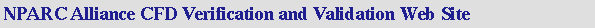V&V Home       Archive       Tutorial

## CFD Analysis Process

The general process for performing a CFD analysis is outlined below so as to provide a reference for understanding the various aspects of a CFD simulation. The process includes:

In further detail, these steps include:

1. #### Formulate the Flow Problem

The first step of the analysis process is to formulate the flow problem by seeking answers to the following questions:

• what is the objective of the analysis?
• what is the easiest way to obtain those objective?
• what geometry should be included?
• what are the freestream and/or operating conditions?
• what dimensionality of the spatial model is required? (1D, quasi-1D, 2D, axisymmetric, 3D)
• what should the flow domain look like?
• what is the nature of the viscous flow? (inviscid, laminar, turbulent)
• how should the gas be modeled?

2. #### Model the Geometry and Flow Domain

The body about which flow is to be analyzed requires modeling. This generally involves modeling the geometry with a CAD software package. Approximations of the geometry and simplifications may be required to allow an analysis with reasonable effort. Concurrently, decisions are made as to the extent of the finite flow domain in which the flow is to be simulated. Portions of the boundary of the flow domain conicide with the surfaces of the body geometry. Other surfaces are free boundaries over which flow enters or leaves. The geometry and flow domain are modeled in such a manner as to provide input for the grid generation. Thus, the modeling often takes into account the structure and topology of the grid generation.

3. #### Establish the Boundary and Initial Conditions

Since a finite flow domain is specified, physical conditions are required on the boundaries of the flow domain. The simulation generally starts from an initial solution and uses an iterative method to reach a final flow field solution.

4. #### Generate the Grid

The flow domain is discretized into a grid. The grid generation involves defining the structure and topology and then generating a grid on that topology. Currently all cases involve multi-block, structured grids; however, the grid blocks may be abbuting, contiguous, non-contiguous, and overlapping. The grid should exhibit some minimal grid quality as defined by measures of orthogonality (especially at the boundaries), relative grid spacing (15% to 20% stretching is considered a maximum value), grid skewness, etc... Further the maximum spacings should be consistent with the desired resolution of important features. The resolution of boundary layers requires the grid to be clustered in the direction normal to the surface with the spacing of the first grid point off the wall to be well within the laminar sublayer of the boundary layer. For turbulent flows, the first point off the wall should exhibit a y+ value of less than 1.0.

5. #### Establish the Simulation Strategy

The strategy for performing the simulation involves determining such things as the use of space-marching or time-marching, the choice of turbulence or chemistry model, and the choice of algorithms.

6. #### Establish the Input Parameters and Files

A CFD codes generally requires that an input data file be created listing the values of the input parameters consisted with the desired strategy. Further the a grid file containing the grid and boundary condition information is generally required. The files for the grid and initial flow solution need to be generated.

7. #### Perform the Simulation

The simulation is performed with various possible with options for interactive or batch processing and distributed processing.

8. #### Monitor the Simulation for Completion

As the simulation proceeds, the solution is monitored to determine if a "converged" solution has been obtained, which is iterative convergence. Further discussion can be found on the page entitled Examining Iterative Convergence.

9. #### Post-Process the Simulation to get the Results

Post-Processing involves extracting the desired flow properties (thrust, lift, drag, etc...) from the computed flowfield.

10. #### Make Comparisons of the Results

The computed flow properties are then compared to results from analytic, computational, or experimental studies to establish the validity of the computed results.

11. #### Repeat the Process to Examine Sensitivities

The sensitivity of the computed results should be examined to understand the possible differences in the accuracy of results and / or performance of the computation with respect to such things as:

• dimensionality
• flow conditions
• initial conditions
• marching strategy
• algorithms
• grid topology and density
• turbulence model
• chemistry model
• flux model
• artificial viscosity
• boundary conditions
• computer system

Further information can be found on the pages entitled Verification Assessment and Validation Assessment.

12. #### Document

Documenting the findings of an analysis involves describing each of these steps in the process.

Last Updated: Wednesday, 10-Feb-2021 09:38:59 EST